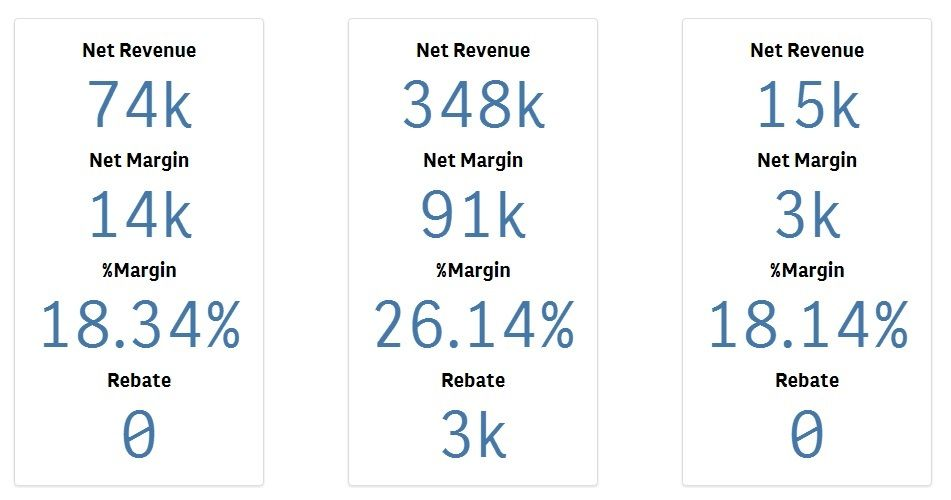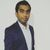# App Development

Discussion board where members can learn more about Qlik Sense App Development and Usage.

Announcements
Welcome to Qlik Community! Check out our new navigation! FIND OUT MORE
cancel
Showing results for
Did you mean:Partner - Contributor III

## Using Qlik to calculate rebate with condition

Hello Qlik community, I have an issue with rebate app that I have tried to developed. I created measure with if-else condition but it work only with some result. My if-else statement is this one

``````If(Wildmatch(VDR1, 'QLIK*'),
If(Sum({<VDR1 = {"QLIK*"}>}[Net Margin (Performance Sales/PM)])/Sum({<VDR1 = {"QLIK*"}>}[Net Revenue (Performance)]) > 0.12,
If(Sum({<VDR1 = {"QLIK*"}>}[Net Revenue (Performance)]) >= 1000000, Sum({<VDR1 = {"QLIK*"}>}[Net Revenue (Performance)])*1.5/100,
If(Sum({<VDR1 = {"QLIK*"}>}[Net Revenue (Performance)]) >= 300000, Sum({<VDR1 = {"QLIK*"}>}[Net Revenue (Performance)])/100, 0)
)
),0
)``````

So I used this one with the data and result look like thisIt works fine with some result but in the red box the Revenue up to 407k so it should be go into the condition like the 348k but its show Rebate as 0. What do I miss in my if-else statement or any idea?

1 Solution

Accepted SolutionsPartner - Contributor III
Author

I can fix this issue by using set analysis instead of using WildMatch. Thank you for your advice.

9 RepliesPartner - Contributor III
Author

I have just found the cause of the problem. It happened because there is more than one Qlik product for example: Qlik - A, Qlik - B, Qlik - C, etc. So when it's come to calculation in Rebate application if data show customers sales Qlik - A and Qlik - B, it will not calculate like in if-else statement. It will calculate only one Qlik product like in 348k they sales only Qlik - A application will work fine but for 407k they sales Qlik - A for 200k and Qlik - B for 207k . That makes Rebate come out as 0.

Any idea for fixing this issue? Any advice and suggestions will be greatly appreciated.Master

Hi

Not able to understand the cause of the problemPartner - Contributor III
Author

Sorry I will give you the example table of this data

 VDR1 Customers Sales Margin QLIK - A A 387000 120000 QLIK - B B 100000 23000 QLIK - D C 200000 100000 QLIK - A D 200000 50000 QLIK - B D 207000 55000 QLIK - C E 10000 2300 QLIK - A F 30000 12000 QLIK - C G 4000000 1200000

From this table up there customers A, D, and G should get rebate because minimum to get rebate in statement is 300000. But this Rebate application will calculate rebate only customer A and G.

For customer D sales QLIK - A and QLIK - B the application should use sale of A and B combine and calculate rebate but it calculate them separately so the result will come out as 0 because QLIK - A and QLIK - B is not above 300000.

Hope this one clearer than last of my reply.Master

Got your point, thanks.

Then why are you considering the first Column while calculating rebate?Let it be only Customer, Sales and Margin only!Partner - Contributor III
Author

Thank you for reply. Actually it has more than just Qlik product so each product has its own condition to get rebate. For example, Qlik has to sales more than 300k to get rebate and product X has to sales up to 70k to get rebate.

So, I have to consider the first Column.Partner - Contributor III
Author

I can fix this issue by using set analysis instead of using WildMatch. Thank you for your advice.MVP

Issue is here

your values is greater than 1000K so it validating under the first condition itself so add an and condition like below else change the order of 300k and 100k

If(Sum({<VDR1 = {"QLIK*"}>}[Net Margin (Performance Sales/PM)])>=1000000 and  Sum({<VDR1 = {"QLIK*"}>}[Net Margin (Performance Sales/PM)])<3000000,  Formula )

```If(Wildmatch(VDR1, 'QLIK*'),
If(Sum({<VDR1 = {"QLIK*"}>}[Net Margin (Performance Sales/PM)])/Sum({<VDR1 = {"QLIK*"}>}[Net Revenue (Performance)]) > 0.12,
If(Sum({<VDR1 = {"QLIK*"}>}[Net Revenue (Performance)]) >= 1000000, Sum({<VDR1 = {"QLIK*"}>}[Net Revenue (Performance)])*1.5/100,
If(Sum({<VDR1 = {"QLIK*"}>}[Net Revenue (Performance)]) >= 300000, Sum({<VDR1 = {"QLIK*"}>}[Net Revenue (Performance)])/100, 0)
)
),0
)```Master

Yes, that is a good idea too.Partner - Contributor III
Author

Thank you for your advice but I try it and result still the same.

``````If(Sum({<VDR1 = {"QLIK*"}>}[Net Margin (Performance Sales/PM)])/Sum({<VDR1 = {"QLIK*"}>}[Net Revenue (Performance)]) > 0.12,
If(Sum({<VDR1 = {"QLIK*"}>}[Net Revenue (Performance)]) >= 1000000,
Sum({<VDR1 = {"QLIK*"}>}[Net Revenue (Performance)])*1.5/100,
If(Sum({<VDR1 = {"QLIK*"}>}[Net Revenue (Performance)]) >= 300000,
Sum({<VDR1 = {"QLIK*"}>}[Net Revenue (Performance)])/100,
0
)
)
)``````

This one is the one make issue fixed.Tags
Community Browser